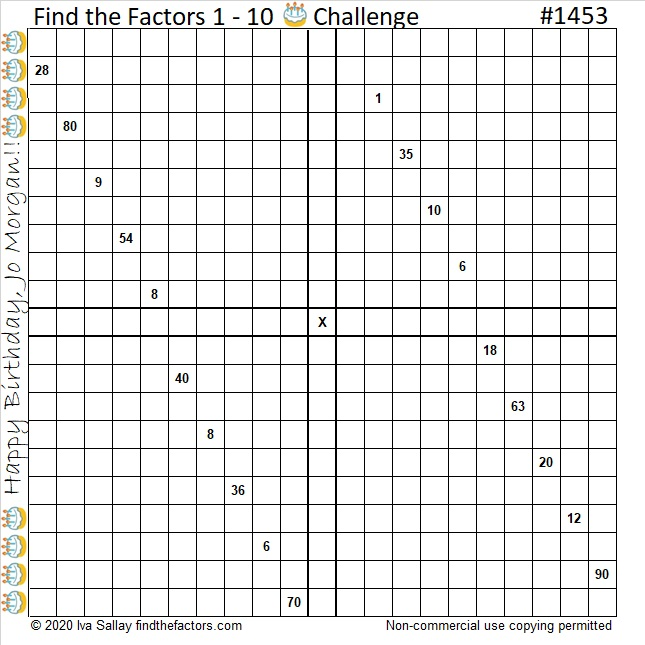# 1453 Happy Birthday, Jo Morgan

Contents

#### Who Is Jo Morgan?

Jo Morgan is an inspiring mathematics teacher, collaborator, tweeter, blogger, podcast guest, and author. Today is her birthday!

Jo recently published her first book, A Compendium of Mathematical Methodsand it is all the rage on twitter. Here is a small sampling of tweets expressing excitement for her book:

I can hardly wait until February 4th when Amazon makes it available in the United States!

Jo has enjoyed solving some of my puzzles, so to commemorate her birthday, I’ve made one especially for her. To solve this puzzle, write the numbers 1 to 10 in each of the four sections outlined in bold so that those numbers are the factors of the product clues given in each of the four mixed-up multiplication tables that make up the puzzle. Use logic to solve the puzzle, but I’m warning you, it won’t be easy.

Happy birthday, Jo! I hope you enjoy the puzzle!

#### Find the Factors 1 – 10 Birthday Challenge Puzzle:Print the puzzles or type the solution in this excel file: 12 Factors 1443-1453

#### Factors of 1453:

It is convenient for puzzles to be numbered, and this puzzle number is 1453. Here are a few facts about that number:

• 1453 is a prime number.
• Prime factorization: 1453 is prime.
• 1453 has no exponents greater than 1 in its prime factorization, so √1453 cannot be simplified.
• The exponent in the prime factorization is 1. Adding one to that exponent we get (1 + 1) = 2. Therefore 1453 has exactly 2 factors.
• The factors of 1453 are outlined with their factor pair partners in the graphic below.

How do we know that 1453 is a prime number? If 1453 were not a prime number, then it would be divisible by at least one prime number less than or equal to √1453. Since 1453 cannot be divided evenly by 2, 3, 5, 7, 11, 13, 17, 19, 23, 29, 31, or 37, we know that 1453 is a prime number.#### Other Facts about the Number 1453:

1453 is the sum of two squares:
38² + 3² = 1453

That means 1453 is the hypotenuse of a Pythagorean triple:
228-1435-1453 calculated from 2(38)(3), 38² – 3², 38² + 3².

Here’s another way we know that 1453 is a prime number: Since its last two digits divided by 4 leave a remainder of 1, and 38² + 3² = 1453 with 38 and 3 having no common prime factors, 1453 will be prime unless it is divisible by a prime number Pythagorean triple hypotenuse less than or equal to √1453. Since 1453 is not divisible by 5, 13, 17, 29, or 37, we know that 1453 is a prime number.

This site uses Akismet to reduce spam. Learn how your comment data is processed.